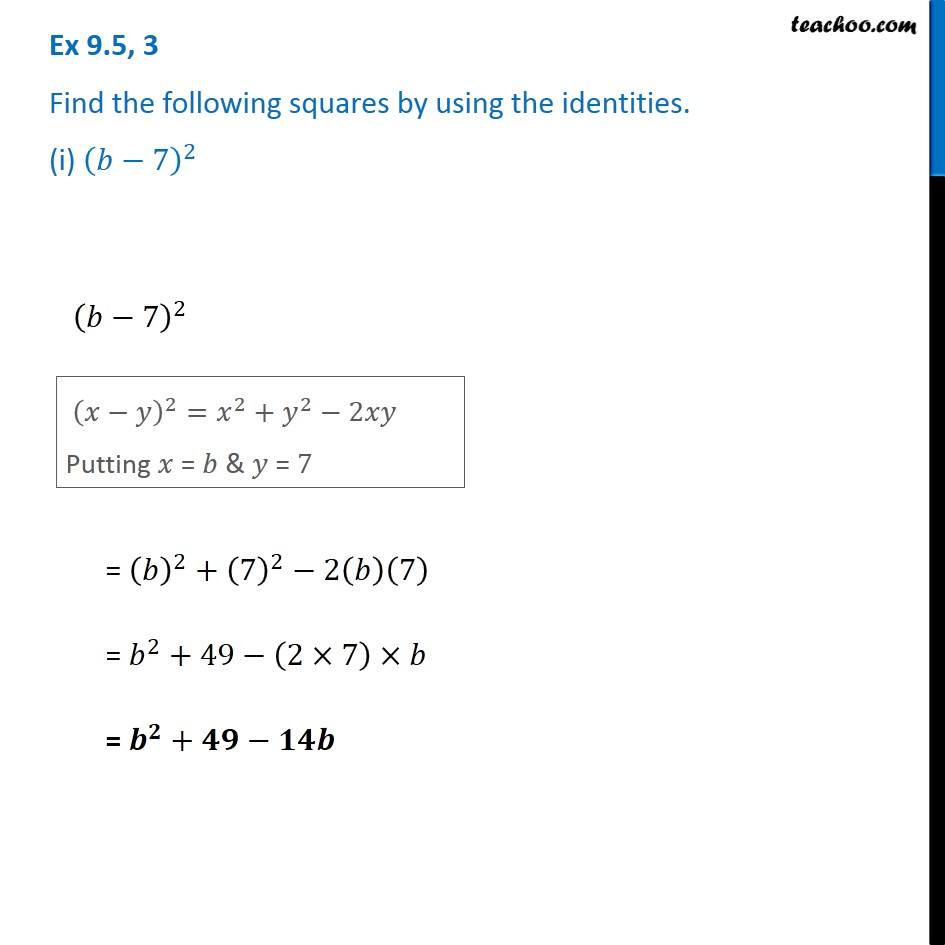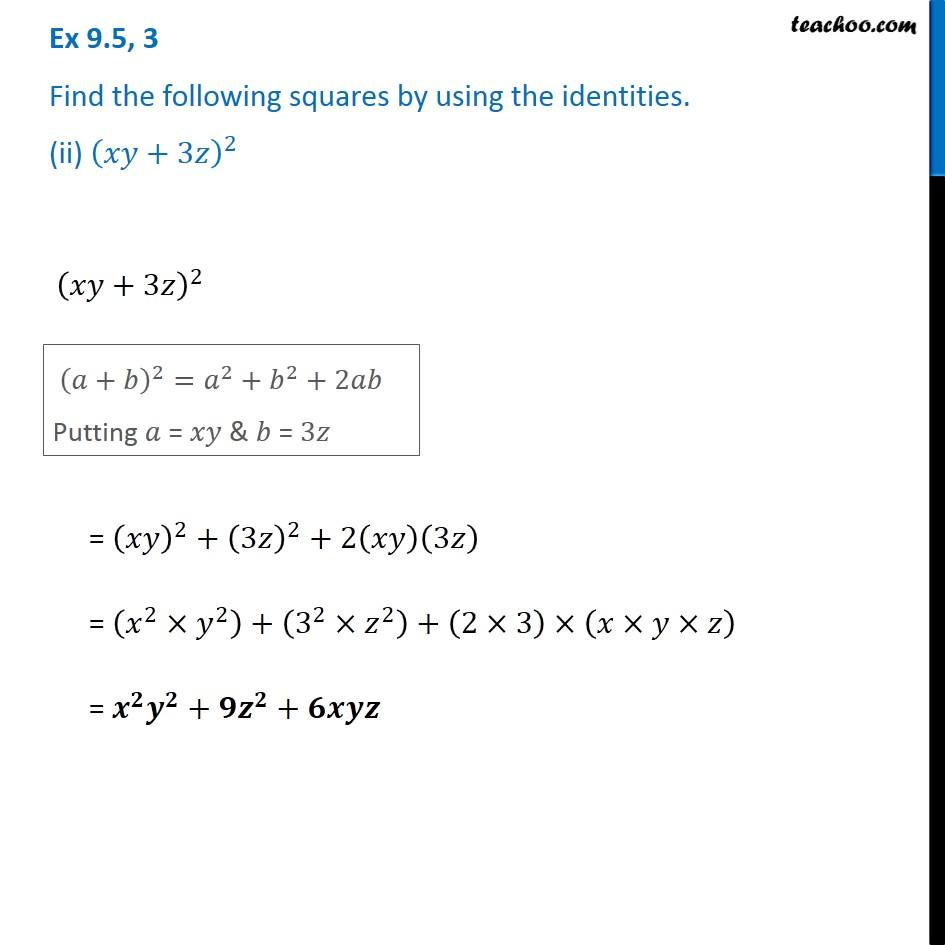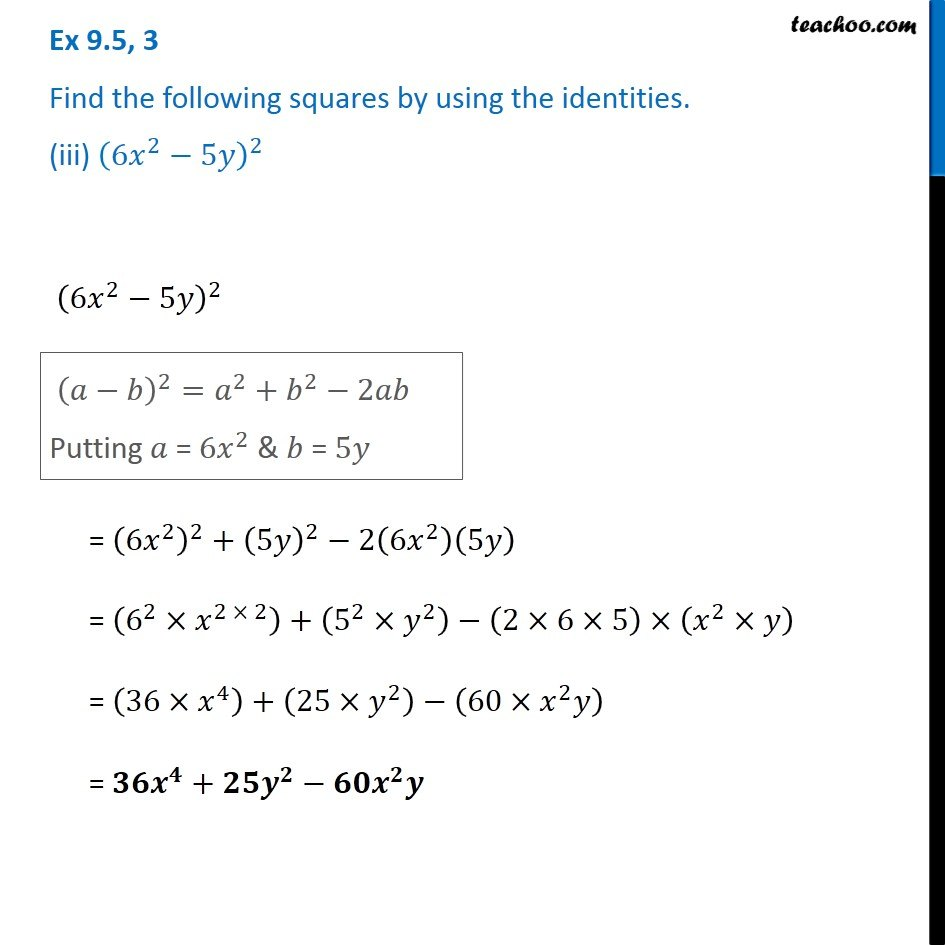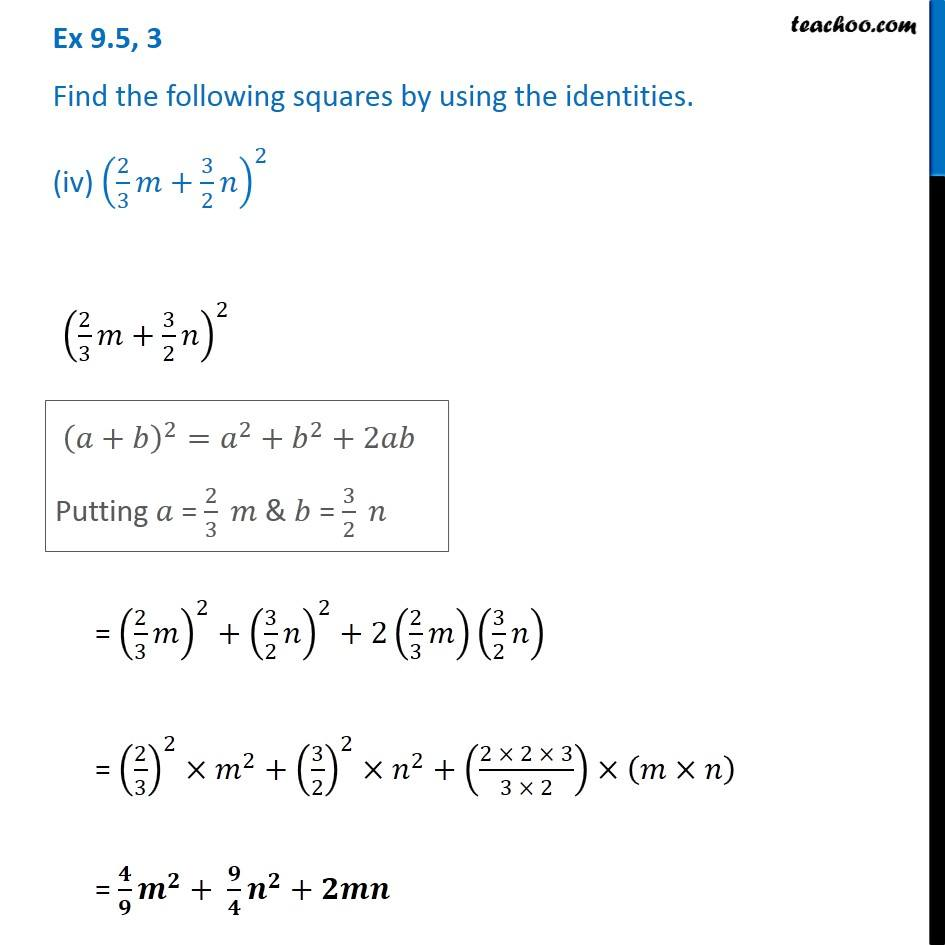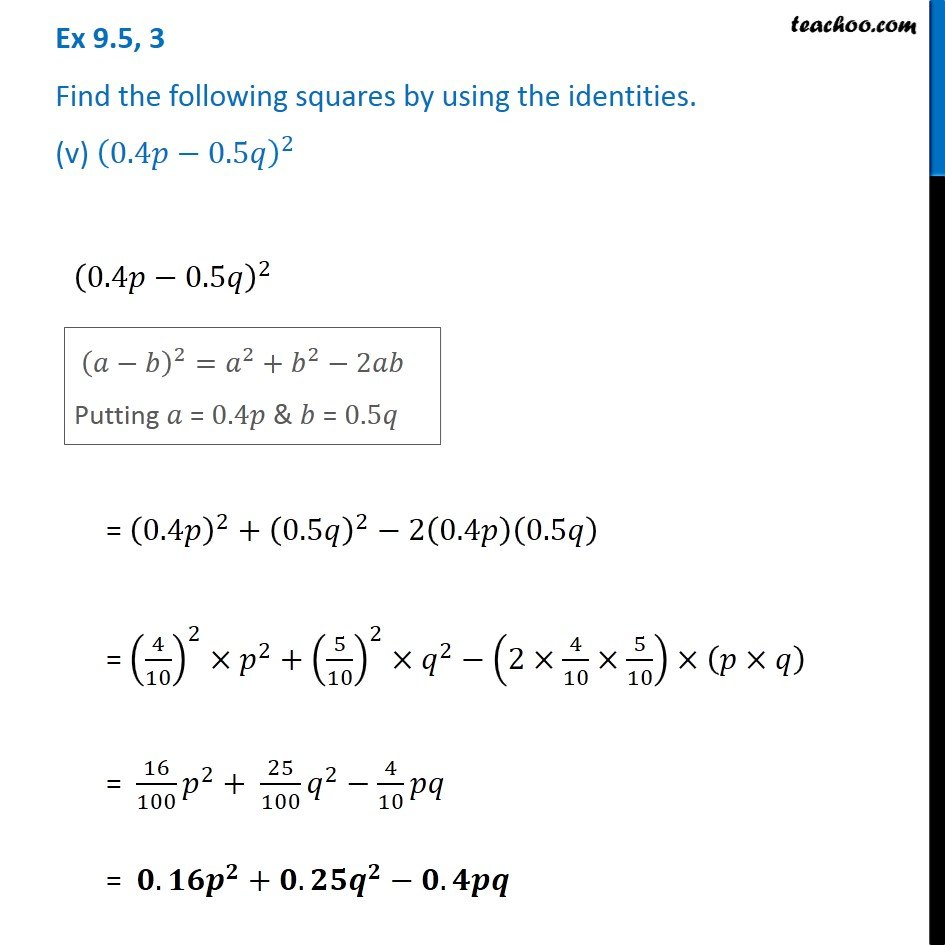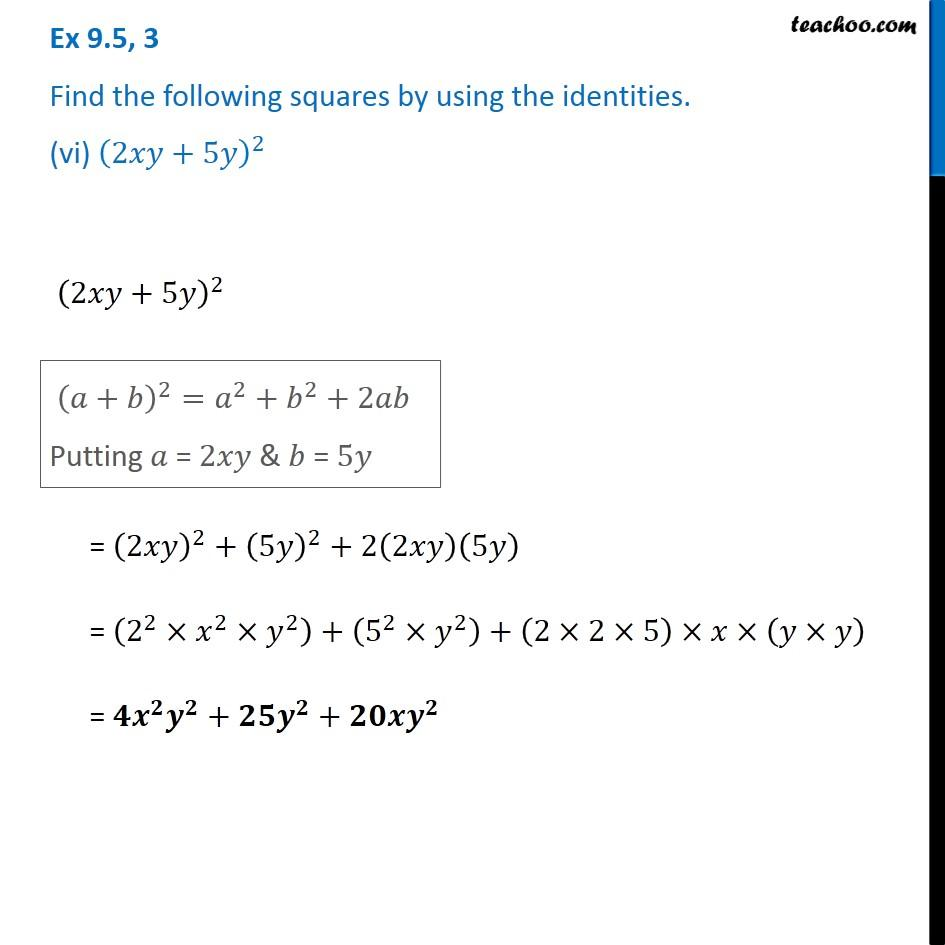1. Chapter 9 Class 8 Algebraic Expressions and Identities
2. Serial order wise
3. Ex 9.5

Transcript

Ex 9.5, 3 Find the following squares by using the identities. (i) (𝑏−7)^2 (𝑏−7)^2 (𝑥−𝑦)^2=𝑥^2+𝑦^2−2𝑥𝑦 Putting 𝑥 = 𝑏 & 𝑦 = 7 = (𝑏)^2+(7)^2−2(𝑏)(7) = 𝑏^2+49−(2×7)×𝑏 = 𝒃^𝟐+𝟒𝟗−𝟏𝟒𝒃 Ex 9.5, 3 Find the following squares by using the identities. (ii) (𝑥𝑦+3𝑧)^2 (𝑥𝑦+3𝑧)^2 (𝑎+𝑏)^2=𝑎^2+𝑏^2+2𝑎𝑏 Putting 𝑎 = 𝑥𝑦 & 𝑏 = 3𝑧 = (𝑥𝑦)^2+(3𝑧)^2+2(𝑥𝑦)(3𝑧) = (𝑥^2×𝑦^2 )+(3^2×𝑧^2 )+(2×3)×(𝑥×𝑦×𝑧) = 𝒙^𝟐 𝒚^𝟐+𝟗𝒛^𝟐+𝟔𝒙𝒚𝒛 Ex 9.5, 3 Find the following squares by using the identities. (iii) (6𝑥^2−5𝑦)^2 (6𝑥^2−5𝑦)^2 (𝑎−𝑏)^2=𝑎^2+𝑏^2−2𝑎𝑏 Putting 𝑎 = 6𝑥^2 & 𝑏 = 5𝑦 = (6𝑥^2 )^2+(5𝑦)^2−2(6𝑥^2 )(5𝑦) = (6^2×𝑥^(2 × 2) )+(5^2×𝑦^2 )−(2×6×5)×(𝑥^2×𝑦) = (36×𝑥^4 )+(25×𝑦^2 )−(60×𝑥^2 𝑦) = 𝟑𝟔𝒙^𝟒+𝟐𝟓𝒚^𝟐−𝟔𝟎𝒙^𝟐 𝒚 Ex 9.5, 3 Find the following squares by using the identities. (iv) (2/3 𝑚+3/2 𝑛)^2 (2/3 𝑚+3/2 𝑛)^2 (𝑎+𝑏)^2=𝑎^2+𝑏^2+2𝑎𝑏 Putting 𝑎 = 2/3 𝑚 & 𝑏 = 3/2 𝑛 = (2/3 𝑚)^2+(3/2 𝑛)^2+2(2/3 𝑚)(3/2 𝑛) = (2/3)^2×𝑚^2+(3/2)^2×𝑛^2+((2 × 2 × 3)/(3 × 2))×(𝑚×𝑛) = 𝟒/𝟗 𝒎^𝟐+ 𝟗/𝟒 𝒏^𝟐+𝟐𝒎𝒏 Ex 9.5, 3 Find the following squares by using the identities. (v) (0.4𝑝−0.5𝑞)^2 (0.4𝑝−0.5𝑞)^2 (𝑎−𝑏)^2=𝑎^2+𝑏^2−2𝑎𝑏 Putting 𝑎 = 0.4𝑝 & 𝑏 = 0.5𝑞 = (0.4𝑝)^2+(0.5𝑞)^2−2(0.4𝑝)(0.5𝑞) = (4/10)^2×𝑝^2+(5/10)^2×𝑞^2−(2×4/10×5/10)×(𝑝×𝑞) = 16/100 𝑝^2+ 25/100 𝑞^2−4/10 𝑝𝑞 = 𝟎.𝟏𝟔𝒑^𝟐+𝟎.𝟐𝟓𝒒^𝟐−𝟎.𝟒𝒑𝒒 Ex 9.5, 3 Find the following squares by using the identities. (vi) (2𝑥𝑦+5𝑦)^2 (2𝑥𝑦+5𝑦)^2 (𝑎+𝑏)^2=𝑎^2+𝑏^2+2𝑎𝑏 Putting 𝑎 = 2𝑥𝑦 & 𝑏 = 5𝑦 = (2𝑥𝑦)^2+(5𝑦)^2+2(2𝑥𝑦)(5𝑦) = (2^2×𝑥^2×𝑦^2 )+(5^2×𝑦^2 )+(2×2×5)×𝑥×(𝑦×𝑦) = 𝟒𝒙^𝟐 𝒚^𝟐+𝟐𝟓𝒚^𝟐+𝟐𝟎𝒙𝒚^𝟐

Ex 9.5